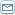# The Art of Hedging in Options Trading

 By: Richard CochraneA hedge is an investment made to offset the risk incurred by entering another investment. Essentially you are setting up a bet on both sides so that one offsets the other and you can end up winning either way.Think of it as a form of insurance.Options are frequently used in hedging. For example, you can speculate that the market price will rise in the future and buy a call today. But, because the market is uncertain and you're not certain it will rise, you simultaneously buy a put option. By carefully selecting the appropriate combinations of strike price, expiration date and type of option an investor can minimize risk and maximize the probability of making a profit.So how does it all work?Well let's take a look at a common hedging strategy: the Strangle. In this strategy, an investor holds both call and put options with the same maturity, but with different strike prices.The contracts are purchased 'out of the money' and are therefore cheaper. 'Out of the money' means the strike price of the underlying asset is higher (for a call) or lower (for a put) than the current market price.For example let's say Intel (INTC) is currently trading at \$40 per share. You could buy one call at \$3 and one put at \$2 with the call having a strike price of \$45, the put \$35. Your total investment would be (\$3 x 100) + (\$2 x 100) = \$500.If the price over the length of the contracts stays between \$35 and \$45 the total possible loss = \$500, the cost of the options. So your risk in this kind of hedge is limited to \$500.Suppose the price drops near expiration to \$25. The call would expire worthless, but the put is worth (\$35-\$25) x 100 = \$1000 - (\$2 x 100) = \$800. Subtract the cost of the call, \$800 - \$300 = \$500. So that's your net profit (ignoring commissions and taxes).The difference between the exposure and the potential profit represents a kind of hedge. Though you are essentially 'betting' that the price could go either way, your downside is limited to the combined cost of the put and the call.There are, not surprisingly, nearly as many hedging strategies as there are investors. A couple of common types are:The collar: Hold the underlying asset and simultaneously both buy a put and sell a call of the same asset. The short call limits gains, but the long put hedges against any losses from the underlying asset.The protective put: Buy the asset and also buy a put option on the same asset. At expiration, the asset may have gained (eliminating the value of the put option), but the rise in the asset offsets the loss.And there are a whole host of other variations. Most do involve speculating on the price direction of the underlying asset, while taking advantage of the leverage, cost and timing characteristics of options. As with any investment strategy, make sure you understand the pros and cons before laying down your bet.